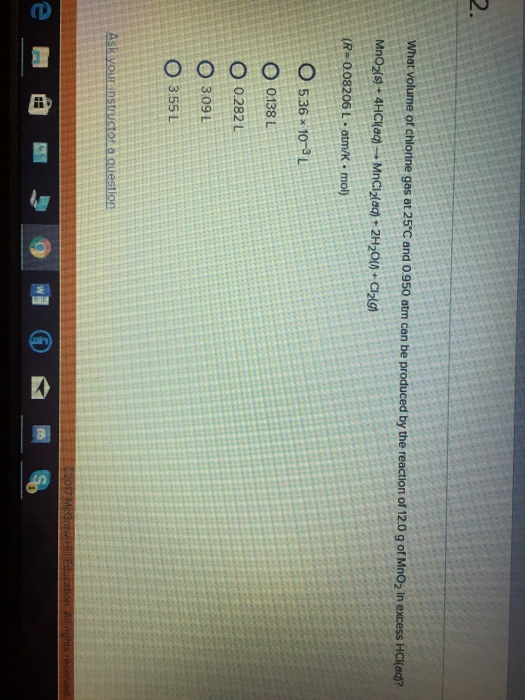# Question & Answer: What volume of chlorine gas at 25 degree C and 0.950 atm can be produced by the reaction of…..What volume of chlorine gas at 25 degree C and 0.950 atm can be produced by the reaction of 12.0 g of MnO_2 in excess HCl(aq)? MnO_2(s) + 4HCl(aq) rightarrow MnCl_2(aq) + 2H_2O(l) + Cl_2(g) (R = 0.08206 L middot atm/k middot mol) 5.36 times 10^-3 L 0.138 L 0.282 L 3.09 L 3.55 L

MnO2 (g) + 4HCl (aq) ————-> MnCl2 (aq) + Cl2(g) + 2H2O(l)

1 mole of MnO2 react with HCl to gives 1 mole of Cl2

Don't use plagiarized sources. Get Your Custom Essay on
Question & Answer: What volume of chlorine gas at 25 degree C and 0.950 atm can be produced by the reaction of…..
GET AN ESSAY WRITTEN FOR YOU FROM AS LOW AS \$13/PAGE

86.94 of MnO2 react with HCl to gives 1 mole of Cl2

12g of MnO2 react with HCl to gives = 1*12/86.94    = 0.138 moles of Cl2

PV = nRT

n = 0.138 moles

P   = 0.95atm

T   = 250C   = 25 + 273 = 298K

R = 0.0821L-atm/mole-K

PV = nRT

V   = nRT/P

= 0.138*0.0821*298/0.95